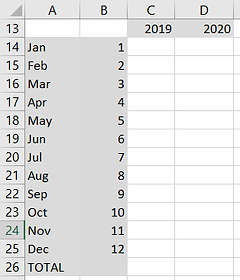## SUMIFS

SUMIFS is a powerful function for summarizing your data.  Below is a simple example to demonstrate the benefit of using this functionality.  Feel free to download my sample data.If you're familiar with pivot tables you know that we can quickly build a pivot table to summarize the orders by month and year.

BUT the pivot table only shows the months with orders.  In addition, every time you refresh your data your columns may change width and formatting.To get started with SUMIFS, I've typed the row and column headers in the desired format for displaying my data.

I've identified both the month number and name, but the month numbers can later be hidden.

If you aren't familiar with using Named Ranges, it will be helpful if you first gain a basic understanding of Named RangesNow we are ready to create the SUMIFS formula.  We want to total the order amounts where the year matches the column header and month matches the month number.

The first parameter in the formula is the sum range followed by the criteria range(s) and the criteria.

In my example for cell D16, we are summing the Amounts, when the the Year column equals the value in row 13 and the Month column equals the value in column B.  =SUMIFS(Amount,Year,D\$13,Month,\$B16)  Learn more about SUMIFSI used the \$ symbol indicates that we want to use a Relative Reference.  This means the row or column should not increment when you copy the formula to other cells.  For example, the formula for April 2020 will be SUMIFS(Amount,Year,D\$13,Month,\$B17).  The rows/columns without a \$ incremented as the formula is copied.  Learn more about relative references

Note if you only have 1 condition you can use SUMIF but I recommend SUMIFS because this function is more flexible and no more difficult to learn.